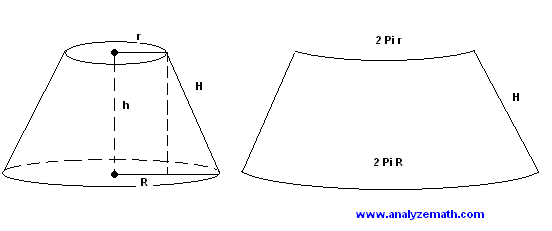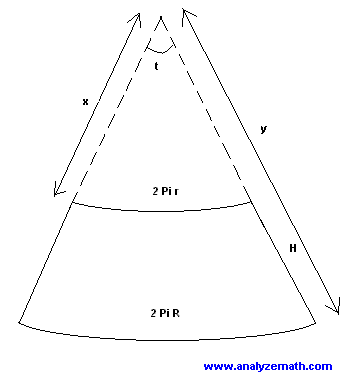# Help with net for a frustum

• Thetom

#### Thetom

I need to make a net for a frustum. The following web page explains how to do it... http://www.analyzemath.com/Geometry/conical_frustum.html

I have tried but am unable to do this complicated, advanced (o.k basic) math.

In the following figure I have the values R,r and h.R = 40
r = 35
h = 83

I need the values for x,y,t and H in the following figure so I can construct my net.x = ?
y = ?
t = ?
H = ?

Last edited:

I need to make a net for a frustum. The following web page explains how to do it... http://www.analyzemath.com/Geometry/conical_frustum.html

I have tried but am unable to do this complicated, advanced (o.k basic) math.

In the following figure I have the values R,r and h.R = 40
r = 35
h = 83

I need the values for x,y,t and H in the following figure so I can construct my net.x = ?
y = ?
t = ?
H = ?

Your internet must not be working properly. If you read on the linked article further, it gives explicit formulas to calculate x, y, and t.

H can be calculated knowing the Pythagorean Theorem:

H2 = h2 + (R-r)2

From the article:

x = r H / (R - r)
y = r H / (R - r) + H

Any of the formulas 2 Pi r = x t or 2 Pi R = y t may be used to find the central angle t (in radians):

t = 2 Pi r / x

= 2 Pi r / [ r H / (R - r) ]

= 2 Pi (R - r) / H

Since the angle t is in radians, to convert t to degrees

t in degrees = t in radians * 180 / Pi

Remember, measure twice, cut once.

Your internet must not be working properly.

No I'm just terrible at this stuff. I did read the solutions given on that page and went through their working example but I still don't understand it.

Here is what I do to solve the Pythagorean Theorem:

H² = h² + (R-r)²

H² = 83x83 + 5x5

H² = 6889 + 25

H² = 6914

H = 1 (6914 / 6914)

I divided the last number by itself as H² is H times by itself. :/
Clearly that's not right but i don't properly understand how to solve the equation or where I'm going wrong.
I think once I have got H i can do the next bit as it seems a bit easier.

I am all for learning how to do this but find it difficult. I appreciate the much needed help.

Remember, measure twice, cut once.

And in my case calculate ten times before asking on PF.

O.k I went ahead and cheated and used an online calculator to solve the Pythagorean problem.
So..

H= 83.15046602394962

I then did this..

x = r H / (R - r)
x = 35 x 83.15 / (40-35)
x = 2910.25 / 5
x = 582.05

y = r H / (R - r) + H
y = 35 x 83.15 / (40 - 35) + 83.15
y = 2910.25 / 5 + 83.15
y = 665.2

t = 2 Pi r / x
t = 2 x 3.141 x 35 / 582.05
t = 219.87 / 582.05
t = 0.38

t in degrees = 0.38 x 180 / Pi
t in degrees = 21.78

So my final values are

H = 83.15
x = 582.05
y = 665.2
t = 21.78°

Is that correct?

O.k I went ahead and cheated and used an online calculator to solve the Pythagorean problem.
So..

H= 83.15046602394962

I then did this..

x = r H / (R - r)
x = 35 x 83.15 / (40-35)
x = 2910.25 / 5
x = 582.05

y = r H / (R - r) + H
y = 35 x 83.15 / (40 - 35) + 83.15
y = 2910.25 / 5 + 83.15
y = 665.2

t = 2 Pi r / x
t = 2 x 3.141 x 35 / 582.05
t = 219.87 / 582.05
t = 0.38

t in degrees = 0.38 x 180 / Pi
t in degrees = 21.78

So my final values are

H = 83.15
x = 582.05
y = 665.2
t = 21.78°

Is that correct?

Your numbers look pretty good. Remember, for H, x, and, y to use consistent measurement units like inches or centimeters when laying out your net.

When you have something like

H2 = 6914

the square root is what tells you how to find H, that is, what number when multiplied by itself, gives you 6914 in this case.

Your calculator has a key that looks like a little check mark √ which is what you press after entering 6914.

When you have something like

H2 = 6914

the square root is what tells you how to find H, that is, what number when multiplied by itself, gives you 6914 in this case.

Your calculator has a key that looks like a little check mark √ which is what you press after entering 6914.

Ah yes that makes sense^
Thanks very much for the help. The page I linked to confused me saying 'sqrt ' before the equations. I get it now. Much appreciated.